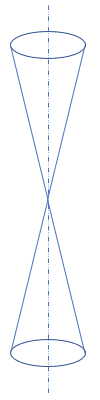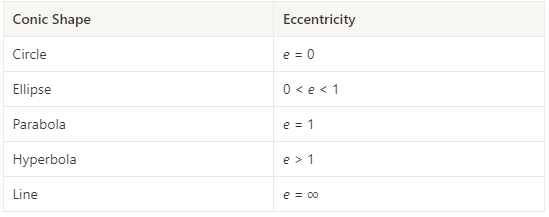## Conic Shapes

Which conic section has an eccentricity equal to one?Hint
The eccentricity, $$e$$ , of a conic section indicates how close its shape is a circle.
Hint 2
The eccentricity of a circle is zero.
The eccentricity, $$e$$ , of a conic section indicates how close its shape is a circle. As eccentricity grows larger, the less the shape resembles a circle. Thus,Parabola